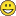# QlikView App Development

Discussion Board for collaboration related to QlikView App Development.

Highlighted
Contributor

## Market Share with calculated dimension

Hi there,

I have a calculated dimension for showing the Top n Corporations + the clients Corporation on a line chart and on a table.

The line chart needs only the Corporations and the Table needs a line with Others.

This is the calculated dimension for the Chart:

IF(

Aggr(

Rank(TOTAL

Sum({<YearMonth ={"\$(=Date(Max(YearMonth), 'MMM-YY'))"},Year=,Month=>}

Value)

), Corporation

) <= vUPLIFT

OR Corporation = 'Client Corporation',

Corporation

)

And this is for the table:

IF(

Aggr(

Rank(TOTAL

Sum({<YearMonth ={"\$(=Date(Max(YearMonth), 'MMM-YY'))"},Year=,Month=>}

Value)

), Corporation

) <= vUPLIFT

OR Corporation = 'Client Corporation',

Corporation,

'Others'

)

The only difference between them is the else 'Others' for the table.

Ok, so I need to calculate the Market Share in both the chart and the table.

In the table works fine, but in the line chart, the total does not consider the Corporations out of the Rank in the calculated dimension, unless I set the dimension to consider null values, but then I'll have always a line in the chart for the null values, which is not what I'm trying to achieveThis is the expression for Market Share calculation:

Value)

/

Sum(TOTAL <YearMonth> {<YearMonth ={">=\$(=Date(AddMonths(Max(YearMonth), -12), 'MMM-YY'))<=\$(=Date(Max(YearMonth), 'MMM-YY'))"},Year=,Month=, Corporation=>}

Value)

So, if I use the calculated dimension with others or accept null values, it shows the proper results. But if disconsider null values using the calculated dimension without others, I'm not able to calculate the total of all Corporations for the right Market Share value.

I need a way to calculate that total per month.

Any ideas?

1 Solution

Accepted SolutionsMVP

## Re: Market Share with calculated dimension

Just use the expression like I suggested, including an outer aggregation and set expression:

Sum({<PeriodMTH ={">=\$(=Date(AddMonths(Max(PeriodMTH), -12), 'MMM-YY'))<=\$(=Date(Max(PeriodMTH), 'MMM-YY'))"}, Period=>}

Soma)

/

Sum({<PeriodMTH ={">=\$(=Date(AddMonths(Max(PeriodMTH), -12), 'MMM-YY'))<=\$(=Date(Max(PeriodMTH), 'MMM-YY'))"}, Period=, Prod=>}

Aggr(NODISTINCT

Sum({<PeriodMTH ={">=\$(=Date(AddMonths(Max(PeriodMTH), -12), 'MMM-YY'))<=\$(=Date(Max(PeriodMTH), 'MMM-YY'))"}, Period=, Prod=>}

Soma)

, PeriodMTH))

5 RepliesMVP & Luminary

## Re: Market Share with calculated dimension

I'm not sure if it led in the right direction but you could try with 1 as set identifier to include the excluded dimension values. Another thought is to calculate your total within a variable which is then independent to your dimension values (they won't be considered and calculate globally) and using them as divisor.

- MarcusMVP

## Re: Market Share with calculated dimension

Using a variable to calculate your market Value in total context might work, but not if you need to get the TOTAL market value across the YearMonth dimension.

Maybe you can use advanced aggregation to get all dimension values into your calculation:

Sum(<YearMonth ={">=\$(=Date(AddMonths(Max(YearMonth), -12), 'MMM-YY'))<=\$(=Date(Max(YearMonth), 'MMM-YY'))"},Year=,Month=, Corporation=>}

Aggr(NODISTINCT

Sum({<YearMonth ={">=\$(=Date(AddMonths(Max(YearMonth), -12), 'MMM-YY'))<=\$(=Date(Max(YearMonth), 'MMM-YY'))"},Year=,Month=, Corporation=>}

Value)

,YearMonth)

)

for your total market value calculation (divisor in the line chart expression).

If this doesn't help, then please post a small sample QVW to have a more detailed look into your setting.

Regards,

Stefan

Contributor

## Re: Market Share with calculated dimension

The formula you proposed brings the correct value, but shows only one month-year.

Attached is an example.MVP

## Re: Market Share with calculated dimension

Just use the expression like I suggested, including an outer aggregation and set expression:

Sum({<PeriodMTH ={">=\$(=Date(AddMonths(Max(PeriodMTH), -12), 'MMM-YY'))<=\$(=Date(Max(PeriodMTH), 'MMM-YY'))"}, Period=>}

Soma)

/

Sum({<PeriodMTH ={">=\$(=Date(AddMonths(Max(PeriodMTH), -12), 'MMM-YY'))<=\$(=Date(Max(PeriodMTH), 'MMM-YY'))"}, Period=, Prod=>}

Aggr(NODISTINCT

Sum({<PeriodMTH ={">=\$(=Date(AddMonths(Max(PeriodMTH), -12), 'MMM-YY'))<=\$(=Date(Max(PeriodMTH), 'MMM-YY'))"}, Period=, Prod=>}

Soma)

, PeriodMTH))

Contributor

## Re: Market Share with calculated dimension

Works like a charm! Thanks a lot!